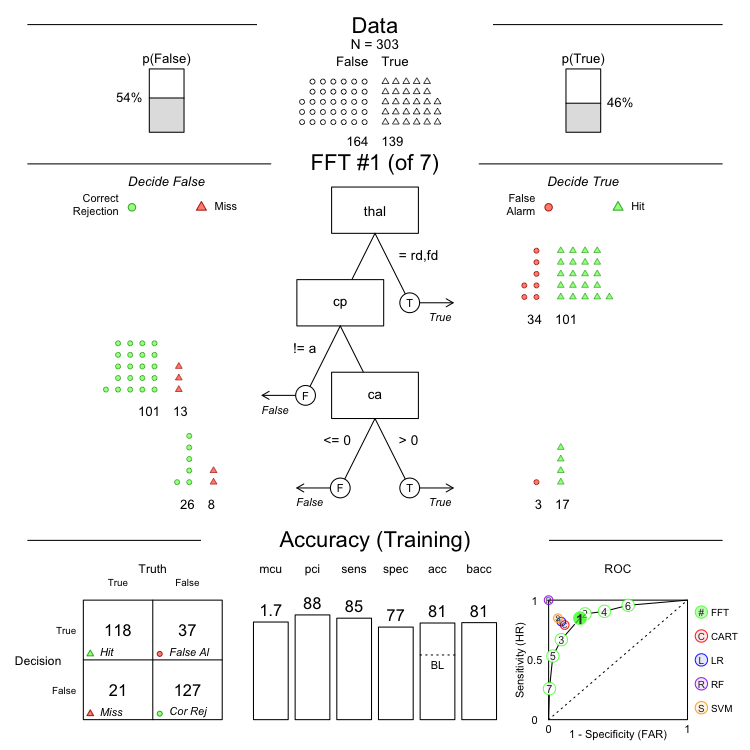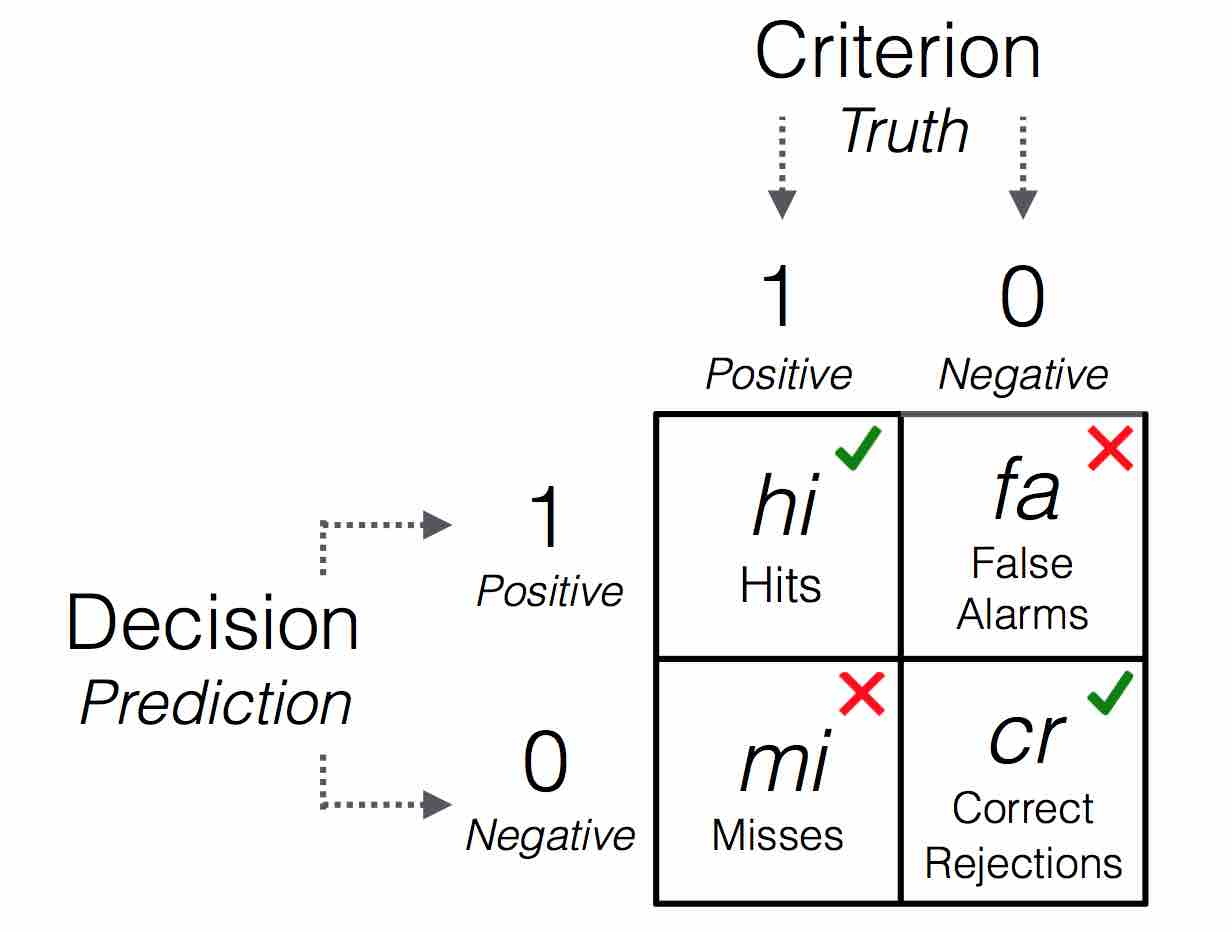# FFTrees Accuracy Statistics

#### 2018-09-15

In this guide, we’ll cover how accuracy statisics are calculated for FFTs. Most of these measures are not specific to FFTs and can be used for any classification algorithm.

First, let’s look at the accuracy statistics from a heart disease FFT:

# Create an FFTrees object predicting heart disease
heart.fft <- FFTrees(formula = diagnosis ~.,
data = heartdisease)

plot(heart.fft)You’ll notice a 2 x 2 table in the bottom-left hand side of the plot. This is a 2 x 2 Confusion Table Wikipedia. All accuracy measures can be derived from this table. Here is a generic version of a confusion table:Confusion table illustrating frequencies of 4 possible outcomes.

The table cross-tabulates the decisions of the algorithm (rows) with actual criterion values (columns) and contains counts of observations for all four resulting cells. Counts in cells a and d refer to correct decisions due to the match between predicted and criterion values, whereas counts in cells b and c refer to errors due to the mismatch between predicted and criterion values. Both correct decisions and errors come in two types: Cell hi represents hits, positive criterion values correctly predicted to be positive, and cell cr represents correct rejections, negative criterion values correctly predicted to be negative. As for errors, cell fa represents false alarms, negative criterion values erroneously predicted to be positive, and cell mi represents misses, positive criterion values erroneously predicted to be negative. Given this structure, an accurate decision algorithm aims to maximize the frequencies in cells hi and cr while minimizing those in cells fa and mi.

Definitions of raw frequencies in a confusion table. The notation $$N()$$ means number of cases.
Output Description Formula
hi Number of hits $$N(Decision = 1 \land Truth = 1)$$)
mi Number of misses $$N(Decision = 0 \land Truth = 1)$$)
fa Number of false-alarms $$N(Decision = 1 \land Truth = 0)$$)
cr Number of correct rejections $$N(Decision = 0 \land Truth = 0)$$)
n Total number of cases $$hi + mi + fa + cr$$

### Conditional accuracy statistics

The first set of accuracy statistics are based on subsets of the data, conditional on either algorithm decisions (positive predictive value and negative predictive value), or criterion values (sensitivity and specificity). In other words, they are based on either rows or columns of the confusion table:

Conditional accuracy statistics based on either rows or columns of the confusion table.
Output Description Formula
sens Sensitivity $$p(Decision = 1 \vert Truth = 1) = hi / (hi + mi)$$
spec Specificity $$p(Decision = 0 \vert Truth = 0) = cr / (cr + fa)$$
far False alarm rate $$1 - spec$$
ppv Positive predictive value $$p(Truth = 1 \vert Decision = 1) = hi / (hi + fa)$$
npv Negative predictive value $$p(Truth = 0 \vert Decision = 0) = cr / (cr + mi)$$

Sensitivity (aka., hit-rate) is defined as $$sens = hi/(hi+mi)$$ and represents the percentage of cases with positive criterion values that were correctly predicted by the algorithm. Similarly, specificity (aka., correct rejection rate, or the compliment of the false alarm rate) is defined as $$spec = cr/(fa + cr)$$ and represents the percentage of cases with negative criterion values correctly predicted by the algorithm.

Positive-predictive value $$ppv$$ and negative predictive value $$npv$$ are the flip-side of $$sens$$ and $$spec$$ as they are conditional accuracies based on decisions (not on true criterion values).

### Aggregate accuracy statistics

The next accuracy statistics are based on all four cells in the confusion table.

Aggreagte accuracy statistics based on all four cells of the confusion table.
Output Description Formula
acc Accuracy $$(hi + cr) / (hi + mi + fa + cr)$$
bacc Balanced accuracy $$sens \times .5 + spec \times .5$$
wacc Weighted accuracy $$sens \times w + spec \times w$$
bpv Balanced predictive value $$ppv \times .5 + npv \times .5$$
dprime D-prime $$zsens - zfar$$

Overall accuracy (acc) is defined as the overall percentage of correct decisions ignoring the difference between hits and correct rejections. $$bacc$$ and $$wacc$$ are averages of sensitivity and specificity, whlie $$bpv$$ is an average of predictive value. $$dprime$$ is the difference in standardized (z-score) transformed $$sens$$ and $$far$$

### Speed and Frugality statistics

The next two statistics measure the speed and frugality of a fast-and-frugal tree. Unlike the accuracy statistics above, they are not based on the confusion table. Rather, they depend on how much information the trees use to make decisions.

Output Description Formula
mcu Mean cues used: Average number of cue values used in making classifications, averaged across all cases
pci Percentage of cues ignored: Percentage of cues ignored when classifying cases $$N(CuesInData) - mcu$$

To see exactly where these statistics come from, let’s look at the results for heart.fft (FFT #1):

heart.fft
## FFT 1 (of 7) predicts diagnosis using 3 cues: {thal, cp, ca}
##
##  If thal = {rd,fd}, decide True.
##  If cp != {a}, decide False.
##  If ca <= 0, decide False, otherwise, decide True.
##
##                     train test
## cases       .n    303.000   --
## hits        .hi   118.000   --
## misses      .mi    21.000   --
## false al    .fa    37.000   --
## corr rej    .cr   127.000   --
## speed       .mcu    1.733   --
## frugality   .pci    0.876   --
## cost        .cost   0.191   --
## accuracy    .acc    0.809   --
## balanced    .bacc   0.812   --
## sensitivity .sens   0.849   --
## specificity .spec   0.774   --
##
## pars: algorithm = 'ifan', goal = 'wacc', goal.chase = 'wacc', sens.w = 0.5, max.levels = 4

According to this output, FFT #1 has $$mcu = 1.73$$ and $$pci = 0.88$$. You can easily calculate these measures directly from the x$levelout output from an FFTrees object. This object contains the level (i.e., node) where each case was classified: # A vector of nodes at which each case was classified in FFT #1 heart.fft$levelout$train[,1] ##  1 3 1 2 2 2 3 3 1 1 1 2 1 1 1 2 1 3 2 2 2 2 2 1 1 2 2 2 3 1 2 1 2 1 2 ##  3 1 1 1 2 1 1 2 2 3 1 2 1 2 2 2 1 3 2 1 1 1 1 2 2 1 2 1 2 1 1 2 1 1 2 ##  2 1 1 1 3 2 1 2 2 1 3 3 2 1 2 2 2 2 3 2 3 1 1 2 2 1 1 1 2 3 3 2 3 2 1 ##  1 1 1 1 1 1 3 1 1 1 1 2 3 1 1 1 1 2 1 2 2 1 1 2 3 1 1 2 3 2 2 1 1 1 2 ##  2 1 2 1 1 2 1 2 2 2 1 3 1 1 3 3 1 1 1 1 1 3 2 3 2 1 2 2 1 2 1 1 3 3 1 ##  1 1 1 2 2 1 1 2 1 3 2 1 1 1 1 2 1 1 3 2 3 2 3 2 2 3 3 1 1 1 1 1 1 2 3 ##  2 1 2 1 3 1 2 3 3 3 2 2 2 1 3 2 3 2 3 3 2 3 2 2 2 3 1 1 2 2 2 2 3 2 2 ##  3 1 3 1 2 1 1 1 2 3 2 3 2 2 1 2 2 2 2 3 1 3 1 1 2 1 1 1 3 2 1 2 2 2 3 ##  1 2 1 2 1 1 1 1 1 2 1 2 1 1 3 2 1 1 1 1 1 2 2 Now, to calculate $$mcu$$ (mean cues used), we simply take the mean of this vector: # Calculate the mean (this is mcu) mean(heart.fft$levelout$train[,1]) ##  1.73 Now that we know where $$mcu$$ comes from, $$pci$$ is easy: it’s just the total number of cues in the dataset minus $$mcu$$ divided by the total number of cues in the data: # Calculate pci (percent cues ignored) directly: # (N.Cues - mcu) / (N.Cues) (ncol(heartdisease) - heart.fft$tree.stats$train$mcu) / ncol(heartdisease)
##  0.876

### Cost statistics

Cost statistics are generated by sum of outcomes times user specified costs for those outcomes:

Output Description Formula
cost Algorithm cost $$hi \times cost_{hi} + mi \times cost_{mi} + fa \times cost_{fa} + cr \times cost_{cr}$$